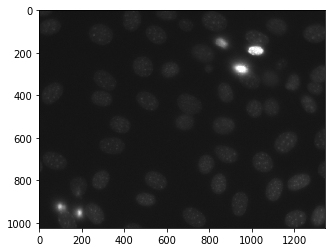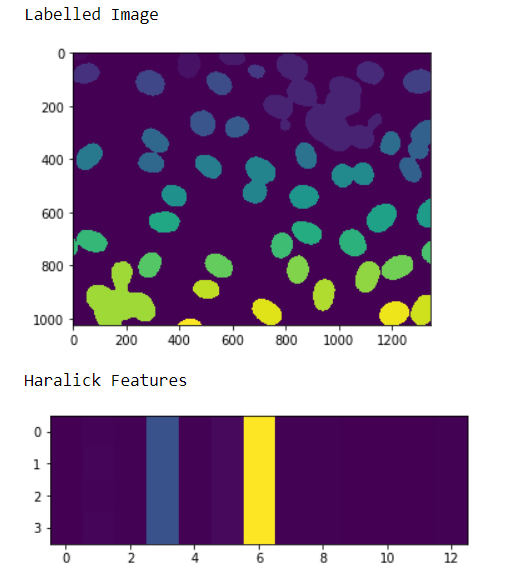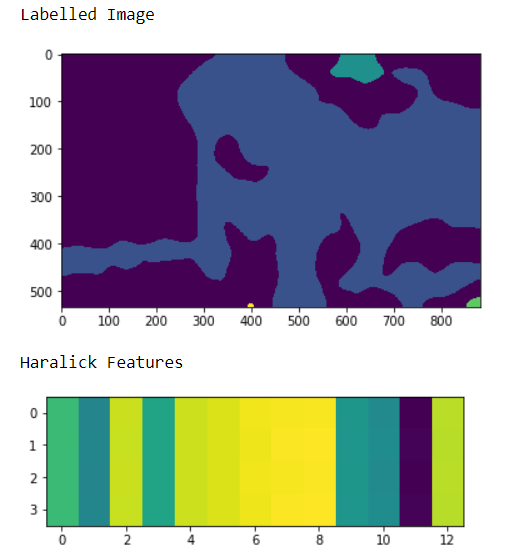Related Articles

# Mahotas – Haralick features

• Difficulty Level : Easy
• Last Updated : 30 Jun, 2021

In this article we will see how we can get the haralick features of image in mahotas. Haralick texture features are calculated from a Gray Level Co-occurrence Matrix, (GLCM), a matrix that counts the co-occurrence of neighboring gray levels in the image. The GLCM is a square matrix that has the dimension of the number of gray levels N in the region of interest (ROI). For this we are going to use the fluorescent microscopy image from a nuclear segmentation benchmark. We can get the image with the help of command given below

`mahotas.demos.nuclear_image()`

Below is the nuclear_imageIn order to do this we will use mahotas.features.haralick method

Syntax : mahotas.features.haralick(img)
Argument : It takes image object as argument
Return : It returns numpy.ndarray

Note : The input of the this should should be the filtered image or loaded as grey
In order to filter the image we will take the image object which is numpy.ndarray and filter it with the help of indexing, below is the command to do this

`image = image[:, :, 0]`

Example 1 :

## Python3

 `# importing various libraries``import` `mahotas``import` `mahotas.demos``import` `mahotas as mh``import` `numpy as np``from` `pylab ``import` `imshow, show` `# loading nuclear image``nuclear ``=` `mahotas.demos.nuclear_image()` `# filtering image``nuclear ``=` `nuclear[:, :, ``0``]` `# adding gaussian filter``nuclear ``=` `mahotas.gaussian_filter(nuclear, ``4``)` `# setting threshold``threshed ``=` `(nuclear > nuclear.mean())` `# making is labeled image``labeled, n ``=` `mahotas.label(threshed)` `# showing image``print``(``"Labelled Image"``)``imshow(labeled)``show()` `# getting haralick features``h_feature ``=` `mahotas.features.haralick(labelled)` `# showing the feature``print``(``"Haralick Features"``)``imshow(h_feature)``show()`

Output :Example 2 :

## Python3

 `# importing required libraries``import` `numpy as np``import` `mahotas``from` `pylab ``import` `imshow, show`` ` `# loading image``img ``=` `mahotas.imread(``'dog_image.png'``)``   ` `# filtering the image``img ``=` `img[:, :, ``0``]``    ` `# setting gaussian filter``gaussian ``=` `mahotas.gaussian_filter(img, ``15``)`` ` `# setting threshold value``gaussian ``=` `(gaussian > gaussian.mean())`` ` `# making is labelled image``labeled, n ``=` `mahotas.label(gaussian)` `# showing image``print``(``"Labelled Image"``)``imshow(labelled)``show()` `# getting haralick features``h_feature ``=` `mahotas.features.haralick(labelled)` `# showing the feature``print``(``"Haralick Features"``)``imshow(h_feature)``show()`

Output :Attention geek! Strengthen your foundations with the Python Programming Foundation Course and learn the basics.

To begin with, your interview preparations Enhance your Data Structures concepts with the Python DS Course. And to begin with your Machine Learning Journey, join the Machine Learning – Basic Level Course

My Personal Notes arrow_drop_up